# Иностранная литература и современность: General Statistics: Ch 5 Quiz Flashcards

Set Details Share
created 9 years ago by GreenHero64
99,104 views
This course is designed to acquaint the student with the principles of descriptive and inferential statistics. Topics will include: types of data, frequency distributions and histograms, measures of central tendency, measures of variation, probability, probability distributions including binomial, normal probability and student's t distributions, standard scores, confidence intervals, hypothesis testing, correlation, and linear regression analysis. This course is open to any student interested in general statistics and it will include applications pertaining to students majoring in athletic training, pre-nursing and business.
updated 9 years ago by GreenHero64
College: First year, College: Second year, College: Third year, College: Fourth year
Subjects:
statistics, mathematics, probability & statistics
Page to share:
Embed this setcancel
COPY
code changes based on your size selection
Size:
X

1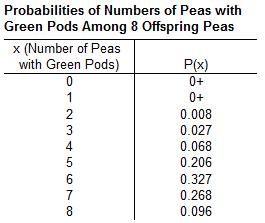The accompanying table describes results from eight offspring peas. The random variable x represents the number of offspring peas with green pods.

1. Find the probability of getting exactly 7 peas with green pods.
2. Find the probability of getting 7 or more peas with green pods.
3. Which probability is relevant for determining whether 7 is an unusually high number of peas with green​ pods, the result from part​ (a) or part​ (b)?
4. Is 7 an unusually high number of peas with green​ pods? Why or why​ not? Use 0.05 as the threshold for an unusual event.

a. 0.268

b. 0.364

(0.268 + 0.096)

c. The result from part (b)

d. No, since the appropriate probability is greater than​ 0.05, it is not an unusually high number.

2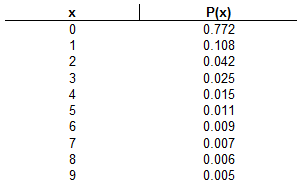Based on data from a car bumper sticker​ study, when a car is randomly​ selected, the number of bumper stickers and the corresponding probabilities are as shown below.

1. Does the given information describe a probability​ distribution?
2. Assuming that a probability distribution is​ described, find its mean and standard deviation.
3. Use the range rule of thumb to identify the range of values for usual numbers of bumper stickers.
4. Is it unusual for a car to have more than one bumper​ sticker? Explain.

a. Yes

b. The mean is 0.6.

The standard deviation is 1.5.

c. The maximum usual value is 3.5.

max: μ + 2σ = 0.578 + 2(1.462161414) = 3.502322828

The minimum usual value is 0.

min: μ – 2σ = 0.578 – 2(1.462161414) = -2.346322828

d. No, because the probability of more than 1 bumper sticker is 0.120​, which is greater than 0.05.

1 – (0.772 + 0.108) = 0.12

3

Determine whether the value is a discrete random​ variable, continuous random​ variable, or not a random variable.

1. The number of free dash throw attempts before the first shot is made
2. The number of light bulbs that burn out in the next week in a room with 18 bulbs
3. The eye color of people on commercial aircraft flights
4. The number of points scored during a basketball game
5. The amount of snowfall in December in City A
6. The number of people in a restaurant that has a capacity of 100

a. It is a discrete random variable.

b. It is a discrete random variable.

c. It is not a random variable.

d. It is a discrete random variable.

e. It is a continuous random variable.

f. It is a discrete random variable.

4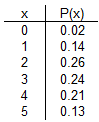In the accompanying​ table, the random variable x represents the number of televisions in a household in a certain country.

Determine whether or not the table is a probability distribution. If it is a probability​ distribution, find its mean and standard deviation.

Its mean is 2.9.

Its standard deviation is 1.3.

5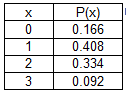Let the random variable x represent the number of girls in a family with three children. Assume the probability of a child being a girl is 0.45. The table on the right describes the probability of having x number of girls.

Determine whether the table describes a probability distribution. If it​ does, find the mean and standard deviation.

Is it unusual for a family of three children to consist of three​ girls?

μ = 1.35

σ = 0.86

No, because the probability of having 3 girls is greater than 0.05.

6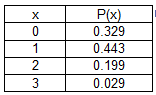Let the random variable x represent the number of girls in a family with three children. Assume the probability of a child being a girl is 0.31. The table on the right describes the probability of having x number of girls.

Determine whether the table describes a probability distribution. If it​ does, find the mean and standard deviation.

Is it unusual for a family of three children to consist of three​ girls?

μ = 0.93

σ = 0.80

Yes, because the probability of having 3 girls is less than or equal to 0.05.

7

In a​ state's Pick 3 lottery​ game, you pay ​\$1.45 to select a sequence of three digits​ (from 0 to​ 9), such as 422. If you select the same sequence of three digits that are​ drawn, you win and collect ​\$439.13.

1. How many different selections are​ possible?
2. What is the probability of​ winning?
3. If you​ win, what is your net​ profit?
4. Find the expected value.
5. If you bet \$ 1.45 in a certain​ state's Pick 4​ game, the expected value is negative \$ 1.01. Which bet is​ better, a ​\$1.45 bet in the Pick 3 game or a \$ 1.45 bet in the Pick 4​ game? Explain.

a. 1,000

b. 0.001

c. \$437.68

(\$439.13 – \$1.45)

d. \$(1.01)

(–\$1.45 + \$439.13/1000)

= -1.45 + 0.44

= -1.01

e. Neither bet is better because both games have the same expected value.

8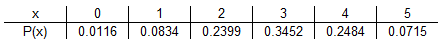Five males with a particular genetic disorder have one child each. The random variable x is the number of children among the five who inherit the genetic disorder.

Determine whether the table describes a probability distribution. If it​ does, find the mean and standard deviation.

μ = 2.9

σ = 1.1

9

Determine whether or not the procedure described below results in a binomial distribution. If it is not​ binomial, identify at least one requirement that is not satisfied.

Six hundred different voters in a region with two major political​ parties, A and​ B, are randomly selected from the population of 3.7 million registered voters. Each is asked if he or she is a member of political party A.

Yes, the result is a binomial probability distribution.

10

A certain TV show recently had a share of 85​, meaning that among the TV sets in​ use, 85​% were tuned to that show. Assume that an advertiser wants to verify that 85​% share value by conducting its own​ survey, and a pilot survey begins with 8 households having TV sets in use at the time of the TV show broadcast.

1. Find the probability that all of the households are tuned to the TV show.
2. Find the probability that exactly 7 households are tuned to the TV show.
3. Find the probability that at least 7 households are tuned to the TV show.
4. If at least 7 households are tuned to the TV​ show, does it appear that the 85​% share value is​ wrong? Why or why​ not?

a. The probability that all of the households are tuned to the TV show is 0.272.

P(x=8) = (0.85)8 = 0.272490525

b. The probability that exactly 7 households are tuned to the TV show is 0.385.

P(x=7) = ( 8 7 ) x (0.85)7 x (0.15)1

= 0.3846925059

c. The probability that at least 7 households are tuned to the TV show is 0.657.

P(x ≥ 7) = P(x=7 or x=8)

= 0.27249 + 0.38469

= 0.65718

d. No, because 7 households tuned to the TV show is not unusually high if the share is 85​%.

11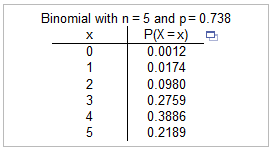Refer to the accompanying technology display. The probabilities in the display were obtained using the values of n = 5 and p = 0.738. In a clinical test of a​ drug, 73.8​% of the subjects treated with 10 mg of the drug experienced headaches. In each​ case, assume that 5 subjects are randomly selected and treated with 10 mg of the drug.

Find the probability that more than one subject experiences headaches.

Is it reasonable to expect that more than one subject will experience​ headaches?

The probability that more than one subject experiences headaches is 0.9814.

P(x > 1) = P(x=2 or x=3 or ... or x=5)

= 1 – P(x=0 or x=1)

= 1 – (0.0012 + 0.0174)

= 0.9814

Yes, because the event that the number of subjects that experience headaches is less than or equal to one is unlikely.

12

Nine peas are generated from parents having the​ green/yellow pair of​ genes, so there is a 0.75 probability that an individual pea will have a green pod.

Find the probability that among the 9 offspring​ peas, at least 8 have green pods.

Is it unusual to get at least 8 peas with green pods when 9 offspring peas are​ generated? Why or why​ not?

The probability that at least 8 of the 9 offspring peas have green pods is 0.300.

P(x ≥ 8) = P(x=8 or x=9)

= [ ( 9 8 ) x (0.75)8 x (0.25)1 ] + [ (0.75)9 ]

= 0.225 + 0.075

= 0.3003387451

No, because the probability of this occurring is not small.

13

A brand name has a 60​% recognition rate. Assume the owner of the brand wants to verify that rate by beginning with a small sample of 5 randomly selected consumers.

1. What is the probability that exactly 4 of the selected consumers recognize the brand​ name?
2. What is the probability that all of the selected consumers recognize the brand​ name?
3. What is the probability that at least 4 of the selected consumers recognize the brand​ name?
4. If 5 consumers are randomly​ selected, is 4 an unusually high number of consumers that recognize the brand​ name?

a. The probability that exactly 4 of the 5 consumers recognize the brand name is 0.259.

P(x=4) = ( 5 4 ) x (0.60)4 x (0.40)1

= 0.2592

b. The probability that all of the selected consumers recognize the brand name is 0.078.

P(x=5) = (0.60)5

= 0.07776

c. The probability that at least 4 of the selected consumers recognize the brand name is 0.337.

P(x ≥ 4) = P(x=4 or x=5)

= 0.259 + 0.078

= 0.33696

d. No​, because the probability that 4 or more of the selected consumers recognize the brand name is greater than 0.05.

14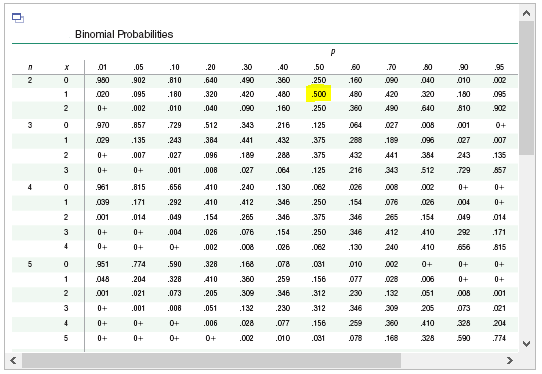Assume that a procedure yields a binomial distribution with n = 2 trials and a probability of success of p = 0.50. Use a binomial probability table to find the probability that the number of successes x is exactly 1.

P(1) = 0.500

15

Assume that a procedure yields a binomial distribution with a trial repeated n times. Use the binomial probability formula to find the probability of x successes given the probability p of success on a single trial.

n=6, x=4, p=0.25

P(4) = 0.033

P(x=4) = ( 6 4 ) x (0.25)4 x (0.75)2

= 0.0329589844

16

Determine whether the given procedure results in a binomial distribution. If it is not​ binomial, identify the requirements that are not satisfied.

Determining whether each of 50 mp3 players is acceptable or defective

Yes, because all 4 requirements are satisfied.

17

A TV​ show, Lindsay and Tobias​, recently had a share of 15​, meaning that among the TV sets in​ use, 15​% were tuned to that show. Assume that an advertiser wants to verify that 15​% share value by conducting its own​ survey, and a pilot survey begins with 18 households having TV sets in use at the time of a Lindsay and Tobias broadcast.

1. Find the probability that none of the households are tuned to Lindsay and Tobias.
2. Find the probability that at least one household is tuned to Lindsay and Tobias.
3. Find the probability that at most one household is tuned to Lindsay and Tobias.
4. If at most one household is tuned to Lindsay and Tobias​, does it appear that the 15​% share value is​ wrong? Why or why​ not?

a. 0.054

P(x=0) = (0.85)18 = 0.0536464098

b. 0.946

P(x ≥ 1) = P(x=1 or x=2 or ... or x=18)

= 1 – P(x=0)

= 1 – 0.054

= 0.9463535902

c. 0.224

P(x ≤ 1) = P(x=0 or x=1)

= 0.054 + [ ( 18 1 ) x (0.15)1 x (0.75)17 ]

= 0.054 + 0.170

= 0.2240526527

d. No, because with a 15% ​rate, the probability of at most one household is greater than 0.05.

18

Determine whether the given procedure results in a binomial distribution. If it is not​ binomial, identify the requirements that are not satisfied.

Treating 150 bald men with a special shampoo and asking them how their scalp feels

No, because there are more than two possible outcomes.

19

Determine whether the given procedure results in a binomial distribution. If it is not​ binomial, identify the requirements that are not satisfied.

Recording the genders of 150 people in a statistics class

Yes, because all 4 requirements are satisfied.

20

Assume that a procedure yields a binomial distribution with n trials and the probability of success for one trial is p.

Use the given values of n and p to find the mean μ and standard deviation σ.

Also, use the range rule of thumb to find the minimum usual value μ – 2σ and the maximum usual value μ + 2σ.

n = 1550​, p = 3 / 5

μ = 930

μ = np

= (1550)(0.60)

= 930

σ = 19.3

σ = √npq

= √(1550 x 0.60 x 0.40)

= 19.28730152

μ – 2σ = 891.4

μ + 2 σ = 968.6